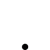Coil Geometry

• Topic Is Sticky
• 150 Views
• Last Post 3 weeks ago
Chris posted this 3 weeks ago

My Friends,

It is easy to take what is obvious to one, for granted, and not realise others may not see this for various different reasons, for this, I apologise!

Coil Geometry is an Important aspect, one which must be taken into account for many reasons! I will try to cover this in more detail in this thread! To fully appreciate the scope of this thread, one must take all aspects into account! Lets start with some fundamentals!

First, I want to point out, most of this has been covered in pretty good detail in my Videos and in various Threads on this Forum.

NOTE: I tried to point this out in the Thread: Delta B in Partnered Output Coils

Voltage V

Pointed out in other Threads, Voltage V is "Generated", predicted by Faradays Law. There are two variations of Faradays Law, each covering two different methods of "Generation" of Voltage V:

• E.M.F = -N dΦb / dt
• E.M.F = Bvl

Electromotive Force, E.M.F is measured in Units of Volts, and is the Total Unit Charge Difference between Two Terminals, one considered Positive and one considered Negative. Electrons e- have a Negative Charge, and a Electron Hole, or Ion e+ not to be confused with the Proton p+, is considered a Positive Charge. As far as Science currently understands, each can "Move" relative to its position in three dimensional Space, relative to the Neiboring Quanta.

The Negative Sign - is Lenz's Law addition to Faradays Law, giving the Sine = 180 to the E.M.F. This is a Newtonian Action and Reaction Pair: For every Action there is an Equal and Opposite Reaction!

Number of Turns N is the Turns Multiplier for the Voltage Measured, or the E.M.F. If one Turn had a Difference in Potential of One Volt, then Ten Turns would have a total Difference of Ten Volts.

Change in Magnetic Field dΦb is also a Differential! This is actually quite easy, we must calculate a BMin and a BMax and take the Difference between the two! A Measured Magnetic Field B Minimum might 10 Gauss, and the Magnetic Field B Maximum might be 110 Gauss, so the Difference is: BMax - BMin = 100 Gauss. This means, = 100 Gauss.

Change in Time dt, is also a Differential, a change in time, if we were 10 seconds into a Test Run on the DUT, and we wanted to start a Measurement of the Magnetic Field B within this Duration, or Time Frame, and we stop at 12 Seconds, then, dt is tMax - tMin = 12 Seconds - 10 Seconds = 2 Seconds.

The Velocity v of the Magnetic Field B is the Speed of which the Magnetic Field B is "Moving".

The Length l, of the Conductor that the Magnetic Field B moves across, not to be confused with Coil Length l also, is a different Variable, that the Magnetic Fiend B with Velocity v, or cuts the Conductor Length l

The Magnetic Field B is a unit of Magnetic Field Density, how much Magnetic Field B can fit into the Cross Sectional Area or CSA.

Coil Cross Sectional Area CSA

Cross Sectional Area, CSA or sometimes just A, is a Windowed Variable. It is the Area of a Circle: A = πr2, or Area of a Rectangle, or a Square: Length l x Width w = A, that the Magnetic Flux B can Change inside. This is a really important Aspect and Floyd Sweet referred to this as Aspect Ratio, giving the Cross Sectional Area an:

• Aspect Ratio
• Time Delay

Where each quantity plays a role in the Signal, or the Magnetic Field B's Rate of Change.

Area can only hold a specific Quantity of Magnetic Field B, and the bigger the Cross Sectional Area CSA, the more Magnetic Field B can be accommodated! Floyd Sweet gave us this Gem:

Now let us consider the losses. Copper or Cu I2R losses remain but may be minimized by using wire of larger than usual cross-sectional area.

Doing some Mathematical Experiments, it is easy to see why this becomes an important statement:

Exp 01: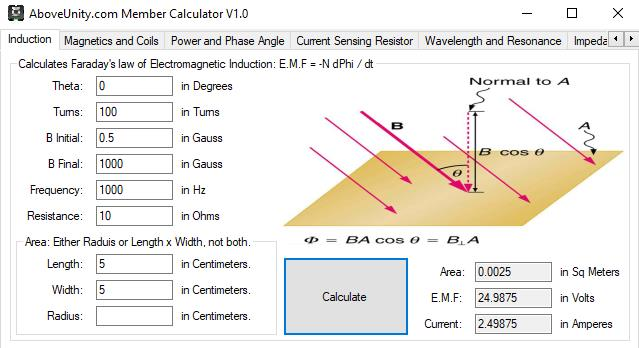Exp 02: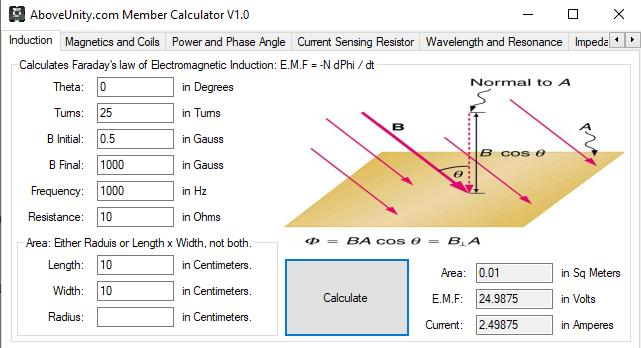By doubling our Area, we have reduced our Turns from 100 to 25, 1/4 the Turns N, and got the same Output Voltage V, and not shown, our reduction in Turns, gives us a much lower Impedance in the Coil!

Coil Length l

Coil Length l, is another very important Variable! Again the Mathematical Experiment is required, and already shown previously, how such a simple change can make such a big difference to the over all Outcome!

Exp 01:Exp 02: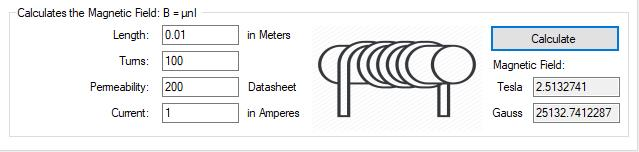By making a single change, to ONLY the Coil Length l, we have: 25132.7412287 / 2513.27412287 = 10 times more Magnetic Field B, for no extra Current I or Turns N! Our Magnetic Field is Stronger for No Cost, only a Geometry Change!

All we have done is make our Coils Shorter in Coil Length l by a Factor of 10. From 0.1 to 0.01 Meters, or 1Cm.

Coil Turns N

Coil Turns N gives us many over all Variables, Voltage V, Resistance R, due to Wire Length l and Wire Gauge AWG, Ohms per meter, and other issues like fitting Turns N on specific Spool Size and so on.

The ideal, but not always practical rule should be, minimal Turns N of Maximum Wire Gauge AWG on the shortest possible Spool Length, giving a Short Coil Length l.

So a Large Cross Sectional Area CSA with a Short Coil Length l, and Large Wire Gauge AWG, will give best results for the Output!

NOTE: Wire Gauge AWG is the Opposite of the Gauge Size, 12 Gauge is a thick Wire, 2.052mm, 20 Gauge is 0.8128mm,the Goal should be Thicker than normal Wire, meaning a Lower AWG! The Thicker the Gauge, the Lower the DC Resistance, thus the Goal, reducing the DC Resistance.

In the Electrical Generator, we see this situation all the time: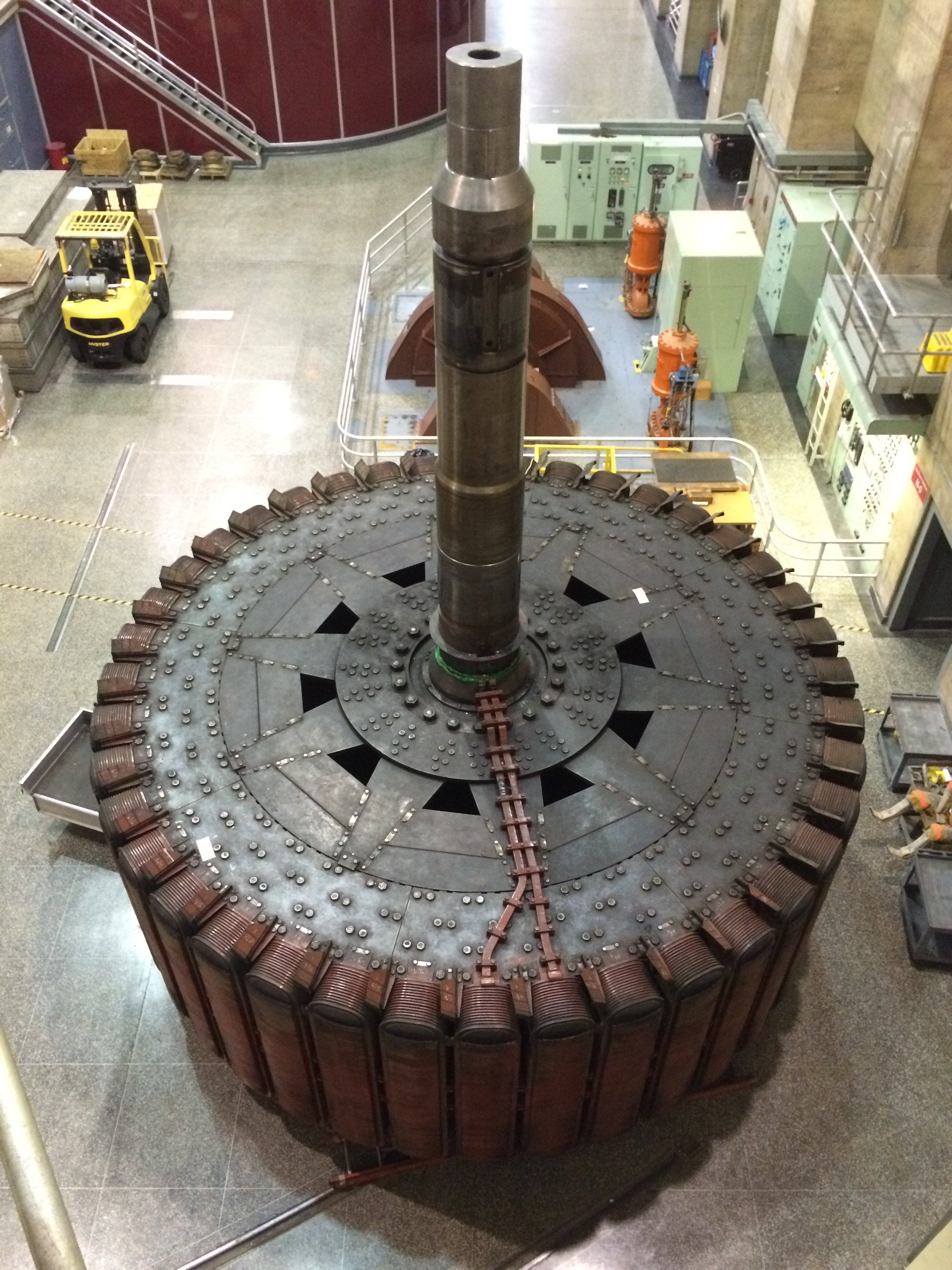A Smart approach to an other wise simple problem, is the only way forward!

My Friends, Magnetic Field BMax is a very important aspect to think about in Faradays Law Equation: E.M.F = -N dΦb / dt, we can see the Larger BMax the more Voltage V "Generated"! Remember I have said, "get your Magnetic Fields Up" this is why.

Partnered Output Coils "Generate" Voltage V and Pump Current I to give: Power P = Voltage V x Current I Cos( Theta θ ) and thus Energy in Joules J is: Joules J = Power P x Time t.

Electrodynamics is entirely Void off Asymmetry, this is why Science is Incomplete!

Best Wishes,

Chris

cd_sharp posted this 3 weeks ago

Hey, buddy

Excellent info! I'd like to clear something for myself. In this screenResistance is actually the entire impedance of the coil (resistance + reactance at the input Frequency), the thing you are talking about above. Is it correct?

Stay strong!

"It's just the knowledge of the coils and how they interact with each other" (Stephen Mark)

Chris posted this 3 weeks ago

Hey CD,

Yes, Impedance Z is the total AC and DC Resistances, and we know AC Resistance is made up of Reactance's XL and XC, both Inductive L and Capacitive C.

At Resonance, there is No AC Resistance, and thus the Impedance Z is just the DC Resistance. This means the Impedance Z = DC Resistance R when the System is in Resonance! We have covered this Here and in other places.

When the Inductive Reactance XL = the Capacitive Reactance XC we have Resonance: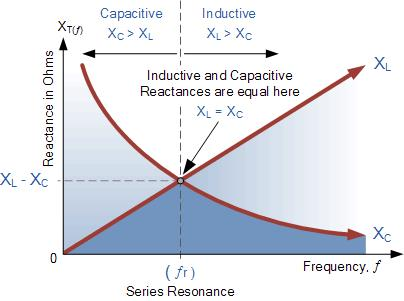So it is still a good idea, to make the DC Resistance R as low as possible, this makes an obvious Improvement seen in the Ohms Law Equation: Current I = Voltage V / Resistance R.

Again, I want to point out, all information in this Thread has been covered many times, all I have done is put it in the one thread as I see so many having trouble with this Subject. For this, I am sorry, I thought most of this was obvious.

Best Wishes,

Chris

Members Online:

No one online at the moment

Since 03.08.17 - Widget: 27.11.18
Recomended Protocol:MeasurementMeasurement BlockCurrent ObservationCurrent Sensing Resolution
Our Above Unity Machines:Romanian ZPMWistiti's Bucking Joule ThiefYo's Ferro-Magnetic ResonanceJagau's Non-linear ResonanceCD's Non-Inductive Coil RepNon-Inductive Coil ExperimentBaerndorfer's ExperimentCaptainloz's A-R-EFerrite at work
Start Here:Help with using the Forum.

More than anything else, your contributions to this forum are most important! We are trying to actively get all visitors involved, but we do only have a few main contributors, which are very much appreciated! If you would like to see more pages with more detailed experiments and answers, perhaps a contribution of another type maybe possible:

PayPal De-Platformed me!

They REFUSE to tell me why!

The content I am sharing is not only unique, but is changing the world as we know it! Please Support Us!

Perhaps a Bank Transfer?

• Bank: Bank of Queensland
• Name: Christopher R Sykes
• BSB: 124-001
• Account: 21580359

Thank You So Much!

Weeks High Earners:
The great Nikola Tesla:Ere many generations pass, our machinery will be driven by a power obtainable at any point of the universe. This idea is not novel. Men have been led to it long ago go by instinct or reason. It has been expressed in many ways, and in many places, in the history of old and new. We find it in the delightful myth of Antheus, who drives power from the earth; we find it among the subtle speculations of one of your splendid mathematicians, and in many hints and statements of thinkers of the present time. Throughout space there is energy. Is this energy static or kinetic? If static, our hopes are in vain; if kinetic - and this we know it is for certain - then it is a mere question of time when men will succeed in attaching their machinery to the very wheelwork of nature.

Experiments With Alternate Currents Of High Potential And High Frequency (February 1892).

Close